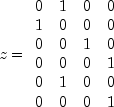JMSLTM Numerical Library 7.2.0
com.imsl.datamining.neural

## Class UnsupervisedNominalFilter

• All Implemented Interfaces:
Serializable

```public class UnsupervisedNominalFilter
extends Object
implements Serializable```
Converts nominal data into a series of binary encoded columns for input to a neural network. It also reverses the aforementioned encoding, accepting binary encoded data and returns an array of integers representing the classes for a nominal variable.

### Binary Encoding

Method `encode` can be used to apply binary encoding. Referring to the result as z, binary encoding takes each category in the nominal variable `x[]`, and creates a column in z containing all zeros and ones. A value of zero indicates that this category was not present and a value of one indicates that it is present.

For example, if `x[]={2, 1, 3, 4, 2, 4}` then `nClasses=4`, andNotice that the number of columns in the result, z, is equal to the number of distinct classes in `x`. The number of rows in z is equal to the length of `x`.

### Binary Decoding

Unfiltering can be performed using the method `decode`. In this case, `z` is the input, and we refer to x as the output. Binary unfiltering takes binary representation in `z`, and returns the appropriate class in x.

For example, if a row in `z` equals {0, 1, 0, 0}, then the return value from `decode` would be 2 for that row. If a row in `z` equals {1, 0, 0, 0}, then the return value from `decode` would be 1 for that row. Notice these are the same values as the first two elements of the original `x[]` because classes are numbered sequentially from 1 to `nClasses`. This ensures that the results of `decode` are associated with the i-th class in `x[]`.

Example, Serialized Form
• ### Constructor Summary

Constructors
Constructor and Description
`UnsupervisedNominalFilter(int nClasses)`
Constructor for `UnsupervisedNominalFilter`.
• ### Method Summary

Methods
Modifier and Type Method and Description
`int` `decode(int[] z)`
Decodes a binary encoded array into its nominal category.
`int[]` `decode(int[][] z)`
Decodes a matrix representing the binary encoded columns of the nominal variable.
`int[]` `encode(int x)`
Apply forward encoding to a value.
`int[][]` `encode(int[] x)`
Encodes class data prior to its use in neural network training.
`int` `getNumberOfClasses()`
Retrieves the number of classes in the nominal variable.
• ### Methods inherited from class java.lang.Object

`clone, equals, finalize, getClass, hashCode, notify, notifyAll, toString, wait, wait, wait`
• ### Constructor Detail

• #### UnsupervisedNominalFilter

`public UnsupervisedNominalFilter(int nClasses)`
Constructor for `UnsupervisedNominalFilter`.
Parameters:
`nClasses` - An `int` specifying the number of categories in the nominal variable to be filtered.
• ### Method Detail

• #### decode

`public int decode(int[] z)`
Decodes a binary encoded array into its nominal category. This is the inverse of the `encode(int)` method.
Parameters:
`z` - An `int` array containing the data to be decoded.
Returns:
An `int` containing the number associated with the category encoded in `z`.
• #### decode

`public int[] decode(int[][] z)`
Decodes a matrix representing the binary encoded columns of the nominal variable. This is the inverse of the `encode(int[])` method.
Parameters:
`z` - An `int` matrix containing the data to be decoded.
Returns:
An `int` array containing the decoded data.
• #### encode

`public int[] encode(int x)`
Apply forward encoding to a value.
Parameters:
`x` - An `int` containing the value to be encoding. Class number must be in the range 1 to `nClasses`.
Returns:
An `int` array containing the encoded data.
• #### encode

`public int[][] encode(int[] x)`
Encodes class data prior to its use in neural network training.
Parameters:
`x` - An `int` array containing the data to be encoded. Class number must be in the range 1 to `nClasses`.
Returns:
An `int` matrix containing the encoded data.
• #### getNumberOfClasses

`public int getNumberOfClasses()`
Retrieves the number of classes in the nominal variable.
Returns:
An `int` containing the number of classes in the nominal variable.
JMSLTM Numerical Library 7.2.0